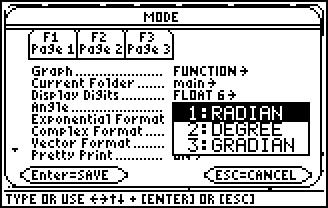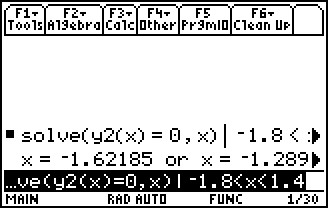# Activities

••• ##### Subject Area

• Math: Calculus: Transcendental Functions
• Math: Calculus: Applications of Integral, Antiderivatives and Definite Integrals
• Math: Calculus: Applications of the Derivative
• Math: Calculus: Derivatives
• Math: Calculus: Antiderivatives and Slope Fields
• Math: Calculus: Limits of Functions

• ##### Author9-12

20 Minutes

• ##### Device
• TI-89 / TI-89 Titanium

## AP Calculus Exam Prep#### Activity Overview

Students prepare for the AP* Calculus exam by reviewing and gaining skills in graphing, finding intersections, zeros, derivatives at a point, and evaluating definite integrals.

*AP, College Board, and SAT are registered trademarks of the College Board, which was not involved in the production of and does not endorse this product.

#### Key Steps

•This activity is written at an introductory level for new or less experienced users of the TI-89 Titanium.

Students will do exercises and learn practice tips to help them prepare for the AP Calculus exam. They also will review and gain skills in graphing, finding intersections, zeros, derivatives at a point and evaluating definite integrals

•In Part 2, examples of non-calculator, multiple-choice questions are provided.